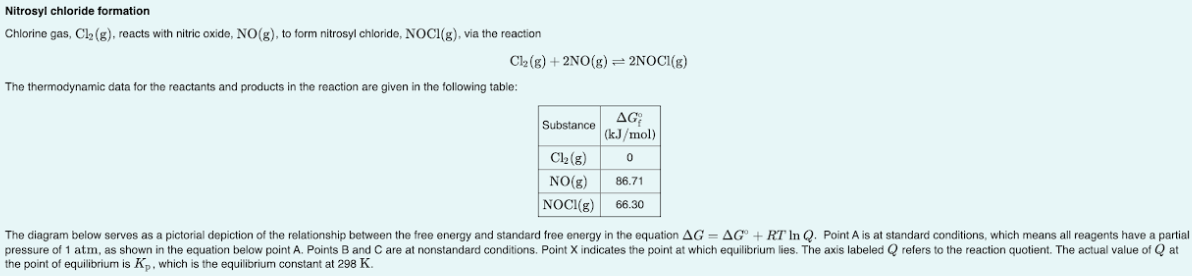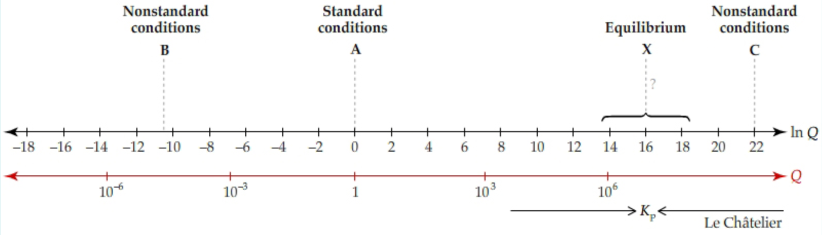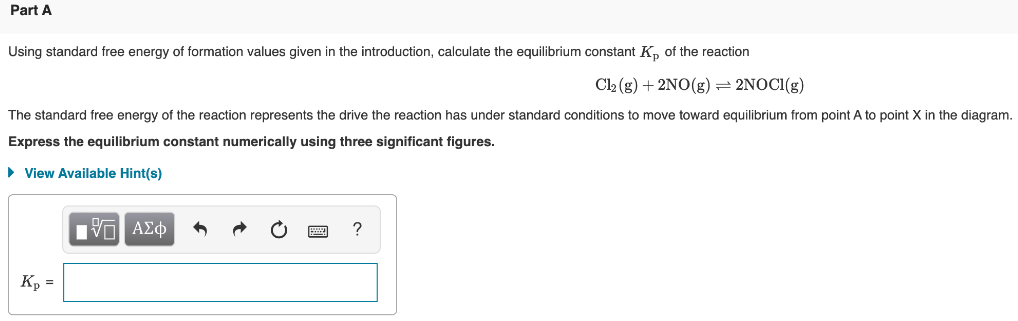# Chlorine gas, Cl2(g), reacts with nitric oxide, NO(g), to form nitrosyl chloride, NOCl(g), via the reaction Cl2(g) + 2NO(g) ⇌ 2NOCl(g) The thermodynamic data for the reactants and products in the reaction are given in the following table: The diagram below serves as a pictorial depiction of the relationship between the free energy and standard free energy in the equation ΔG = ΔG° + RT ln Q. Point A is at standard conditions, which means all reagents have a partial pressure of 1 atm, as shown in the equation below point A. Points B and C are at nonstandard conditions. Point X indicates the point at which equilibrium lies. The axis labeled Q refers to the reaction quotient. The actual value of Q at the point of equilibrium is Kp, which is the equilibrium constant at 298 K. Using standard free energy of formation values given in the introduction, calculate the equilibrium constant Kp of the reaction Cl29g) + 2NO(g) ⇌ 2NOCl(g) The standard free energy of the reaction represents the drive the reaction has under standard conditions to move toward equilibrium from point A to point X n the diagram. Express the equilibrium constant numerically using three significant figures.